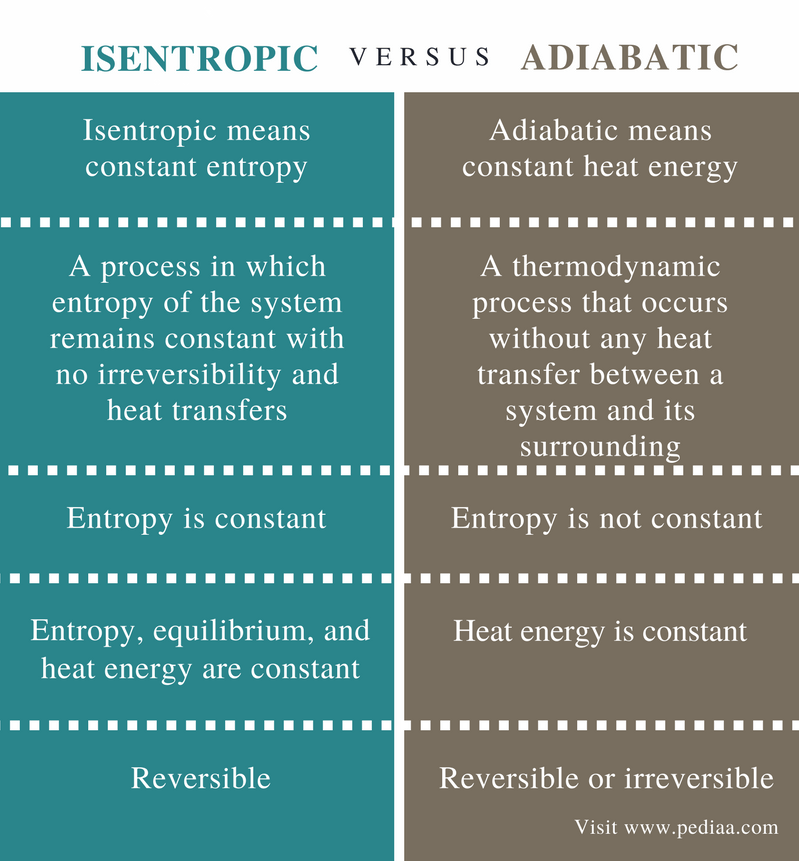# Difference Between Isentropic and Adiabatic

## Main Difference – Isentropic vs Adiabatic

Isentropic and adiabatic are two terms used to name two particular chemical processes that take place in thermodynamic systems. These processes are explained using thermodynamics. Thermodynamics is the branch of physical science that deals with the relations between heat and other forms of energy. Isentropic process is an idealized thermodynamic process. The term isentropic refers to having constant entropy. Therefore, an isentropic process occurs without changing the entropy of the system. On the other hand, adiabatic process is a thermodynamic process in which heat is not either lost or gained by the thermodynamic system. Isentropic process is a type of adiabatic process. The two terms also refer to the system where these processes take place: isentropic system and adiabatic system. The main difference between Isentropic and adiabatic is that isentropic means constant entropy whereas adiabatic means constant heat energy.

### Key Areas Covered

1. What is Isentropic
– Definition, Explanation with Thermodynamics
– Definition, Process, System
3. What are the Similarities Between Isentropic and Adiabatic
– Outline of Common Features
4. What is the Difference Between Isentropic and Adiabatic
– Comparison of Key Differences

Key Terms: Adiabatic, Energy, Entropy, Heat, Isentropic, System, Thermodynamics## What is Isentropic

The term isentropic is used to name either a thermodynamic process or a system where an isentropic process takes place. An isentropic process is a process where entropy of the system remains constant with no irreversibility and heat transfers. This means the entropy of the thermodynamic system remains the same at the end of the process. This process is a type of adiabatic process. It can be explained as a reversible adiabatic process.

An isentropic process holds entropy, equilibrium and heat energy constant. This process is characterized by,

ΔS   =   0   or    S1  =  S2

ΔS is the change in entropy and S1, S2 are initial and final entropies of the system. Some examples of theoretical isentropic systems are pumps, turbines, gas compressors, etc.Figure 1: Entropy is Constant for Isentropic Systems

According to the second law of thermodynamics,

dS  =   dQ/T

dS is change in entropy, dQ is change in heat energy or the heat transfer and T is the temperature. In order to keep a constant entropy, no heat transfer occurs between the system and its surrounding (because according to above the law, increasing the energy increases the entropy) and the work done in the system should be frictionless (friction in the internal system generates entropy).

Adiabatic means constant heat energy, and it can be used to name a thermodynamic process or a system where adiabatic process is taking place. Adiabatic process is a thermodynamic process that occurs without any heat transfer between a system and its surrounding. Here, either heat or matter is not transferred into or out of the system. Therefore, in an adiabatic process, the only way energy transfers between a system and its surrounding is as work.

An adiabatic process can be maintained by quickly doing the process. For example, if we quickly compress a gas in a cylinder, there’s not enough time for the system to transfer heat energy to the environment. In adiabatic processes, the work done by the system changes the internal energy of the system.Figure 2: Adiabatic Reversible State Change

An adiabatic system is a system that has no exchange of energy or matter with the surrounding environment. This means energy is not either lost or gained by the adiabatic system. These systems are known to be adiabatically isolated systems. According to the first law of thermodynamics,

∆U   =    Q   –   W

U is the internal energy of the system, Q is the energy that is exchanged between the system, and it’s surrounding, W is the work done by the system on its surrounding.

For an adiabatic system, Q = 0.

Then,

∆U   =   – W

If we consider a system that is composed of a mixture of gases that act as an adiabatic system when it is expanded, the value of W is positive, and the internal energy is decreased. But if the system contracts, the value of W is negative, and the internal energy is increased. This indicates that energy in an adiabatic process is transferred to its surroundings only as work. Some systems with certain chemical reactions can be approximately considered as adiabatic systems because these reactions happen rapidly, not giving it enough time to release energy outside or gain energy from outside.

## Similarities Between Isentropic and Adiabatic

• Both are thermodynamic processes.
• Isentropic is also a type of adiabatic process.

## Difference Between Isentropic and Adiabatic

### Definition

Isentropic: Isentropic means constant entropy.

### Process

Isentropic: An isentropic process is a process in which entropy of the system remains constant with no irreversibility and heat transfers.

Adiabatic: Adiabatic process is a thermodynamic process that occurs without any heat transfer between a system and its surrounding.

### Entropy

Isentropic: Entropy is constant for isentropic processes or systems.

### Constant Parameters

Isentropic: For isentropic processes or systems, entropy, equilibrium, and heat energy are constant.

### Reversibility

Isentropic: Isentropic processes are reversible.

### Conclusion

The two terms Isentropic and Adiabatic are used to name either thermodynamic processes or systems where those processes take place. The main difference between isentropic and adiabatic is that isentropic means constant entropy whereas adiabatic means constant heat energy.

##### References:

1. “Types of Thermodynamic Process”, Neutrium, Available here.